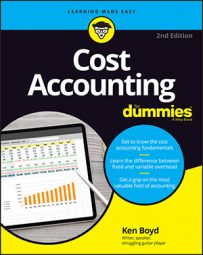##### Cost Accounting For DummiesTo plan your sales and profits in cost accounting, it’s ideal for you to know the breakeven point for every product type you sell. So if you have a store that sells just two product types, compute the breakeven points for both of them.

Say you manage a lumberyard that sells two types of wood to furniture makers: Pristine wood and Sturdy wood. A unit is one board of wood. (If you’re selling first-class hardwoods, the sample prices are pretty close to reality. There’s some serious money in a board 13/16 of an inch thick, 10 inches wide, and 40 inches long.)

Pristine wood is used to make beautiful tabletops and sells for \$50 per unit. Variable costs are \$30 per unit, and fixed costs total \$30,000. The breakeven formula is

Profit (\$0) = sales – variable costs – fixed costs
Profit (\$0) = (units x \$50) – (units x \$30) – \$30,000
Profit (\$0) = units x (\$50 – \$30) – \$30,000
Profit (\$0) = units x \$20 – \$30,000

To finish the calculation, add \$30,000 to both sides of the equation. Then divide both sides by \$20:

\$30,000 = units x \$20
\$30,000 / \$20 = units
1,500 = units

You sell 1,500 units of Pristine wood to break even.

Sturdy wood is used to make pretty good tabletops and sells for \$25 per unit. Variable costs are \$18 per unit, and fixed costs total \$25,000. Here’s the breakeven formula:

Profit (\$0) = sales – variable costs – fixed costs
Profit (\$0) = (units x \$25) – (units x \$18) – \$25,000
Profit (\$0) = units x (\$25 – \$18) – \$25,000
Profit (\$0) = units x \$7 – \$25,000

To finish the calculation, add \$25,000 to both sides of the equation. Then divide both sides by \$7:

\$25,000 = units x \$7
\$25,000 / \$7 = units
3,572 = units

You sell 3,572 units of Sturdy wood to break even.

Pristine wood’s breakeven point in units is less than half of the Sturdy wood’s (1,500 units versus 3,571 units).

A contribution margin of \$20 (Pristine wood) beats a contribution margin of \$7 (Sturdy wood) any day of the week. The difference in fixed costs is \$5,000 — not a big difference. Pristine has a much larger contribution margin to cover fixed costs. That’s why Pristine wood’s breakeven point is so much lower.